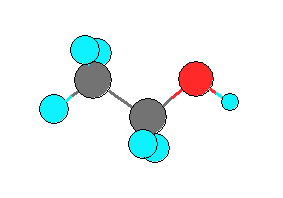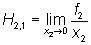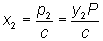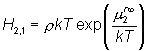# Second Challenge Problem 2

Problem 2 Summary

Determine the Henry’s law constants (HLC) of 4 common gases (N2, CO2, CH4, and O2) in one organic solvent (ethanol [CAS # 64-17-5]) at two temperatures (323 and 373 K).

Ethanol

Hill formula: C2H6O

Other names:
Absolute ethanol
Alcohol
Ethyl alcohol
Ethyl hydroxide
EtOH
Fermentation alcohol
Grain alcohol
MethylcarbinolIntroduction

The solubilities of gases in liquids are properties of great industrial importance since, for example, dissolved gases are a key component of many industrial chemical processes. The inherent experimental difficulties associated with certain gas/solvent mixtures (e.g. the risk of explosion during measurement of oxygen solubility) make them promising candidates for the application of molecular simulation as a tool for physical property prediction.

The Henry’s law constant (HLC) is a measure of the limiting solubility of a solute in a solvent. The HLC of solute 2 in solvent 1 (H2,1 ) at constant temperature can be expressed as(1)
where x2 is the mole fraction of the solute in the solvent, f2 is the fugacity of the solute, and equation (1) is known as Henry’s law. The solubility of a solute in a solvent is often observed to be proportional to the solute’s gas phase partial pressure(2)
as long as the partial pressure is not too large. In equation (2), p2 is the partial pressure of the solute, y2 is the mole fraction of the solute in the gas phase, P is the total pressure, and c is a constant of proportionality. Equations (1) and (2) are consistent if the gas-phase fugacity of the solute is assumed to be equal to it’s partial pressure (i.e. an ideal gas) and c is identified as the Henry’s law constant. A higher Henry's constant value corresponds to a lower solubility.

A number of authors have attempted to use molecular simulation to understand and predict the solubility of a solute in a low molecular weight solvent or in a polymer (e.g. references [2-5]). Historically, the approach most commonly taken has been to estimate the infinite dilution residual chemical potential of the solute in the solvent, , which can be related to Henry’s constant according to(3)
Note that various methods for estimating chemical potential by molecular simulation have recently been discussed. [6,7]

Problem Definition

Determine the Henry’s law constants (HLC) of 4 common gases (N2, CO2, CH4, and O2) in one organic solvent (ethanol [CAS # 64-17-5]) at two temperatures (323 and 373 K). Also specify the uncertainty in the reported values.

Problem Requirements and Restrictions

1. Any force-field parameters (published or unpublished) may be used as long as they do not violate restriction 3 below and are reported in the manuscript submitted to the contest committee.  Any force-field parameters published in a peer-reviewed journal by the date of the Contest announcement may be used, regardless of what experimental data were used to derive them.

2. Any public pure-component data may be used for method or force field development or validation.

3. No data for a mixture of ethanol and one or more of the gases may be used for any purpose. Other public mixture data may be used for method or force field development or validation as long as they have no more than one component (solute or solvent) in common with the contest problem.

Judging Criteria

The Problem 2 numerical score will be a maximum of 100 points:

A) 20 % of the score is based on predicting the correct trend (increasing, decreasing, or nearly constant) with temperature for each gas. “Nearly constant” is defined as an absolute value of the difference between HLC values at the two temperatures that is less than or equal to 10 % of the average of the HLC values at the two temperatures.

B) 40 % of the score is based on a quantitative comparison of the predicted Henry’s law constants with the experimental values, but only in a relative sense. That is, the HLC for each gas at each temperature will be divided by the HLC that is closest to the experimental value at that temperature (in terms of % deviation), and this relative value will be compared to the corresponding relative value based on the experimental data. This is designed to test the relative consistency of the set of calculations as a whole. The values relative to the value closest to experiment are used rather than relative to a particular molecule since otherwise an entry might coincidentally do very poorly for that molecule and score very poorly even if the predictions for the other three were excellent.

C) 40 % of the score is based on a simple quantitative comparison of the predicted Henry’s law constants with the experimental values.

For parts B and C, no credit (0 points) is given if the deviation from experiment is greater than 50 %, full credit is given if the prediction agrees exactly with experiment (within the experimental error), and a prorated number of points is awarded for % deviations less than 50 %.

A spreadsheet is provided on the Contest website which allows any entrant to enter their results and compute the exact numerical score used by the judges.

In order to illustrate how the scoring will be done a Microsoft Excel spreadsheet (evaluation_sheet_2.xls) is provided. The spreadsheet contains pseudo data. The actual experimental data will be available in the spreadsheet after the close of the competition which will allow any entrant to enter their results and compute the exact numerical score used by the judges.

References

1. Prausnitz, J. M., Lichtenthaler, R. N. Azevedo, E. G. d. Molecular Thermodynamics of Fluid-Phase Equilibria (PTR Prentice Hall, Englewood Cliffs, NJ, 1986).

2. Shing, K. S., Gubbins, K. E., Lucas, K. Henry constants in non-ideal fluid mixtures computer-simulation and theory. Mol. Phys. 65, 1235-1252 (1988).

3. Chialvo, A. A. Determination of excess Gibbs free energy by the single-charging-integral approach. 2. Infinite dilution activity coefficients and related quantities. J. Phys. Chem. 95, 6683-6687 (1991).

4. Murad, S., Gupta, S. A simple molecular dynamics simulation for calculating Henry's constant and solubility of gases in liquids. Chem. Phys. Lett. 319, 60-64 (2000).

5. Slusher, J. T. Infinite dilution activity coefficients in hydrogen-bonded mixtures via molecular dynamics: the water/methanol system. Fluid Phase Equilib. 154, 181-192 (1999).

6. Kofke, D. A., Cummings, P. T. Quantitative comparison and optimization of methods for evaluating the chemical potential by molecular simulation. Mol. Phys. 92, 973-996 (1997).

7. Kofke, D. A., Cummings, P. T. Precision and accuracy of staged free-energy perturbation methods for computing the chemical potential by molecular simulation. Fluid Phase Equilib. 150-151, 41-49 (1998).